Courses

# RD Sharma Solutions: Exercise 1.5 & 1.6 - Real Numbers Notes | EduRev

## Class 10 : RD Sharma Solutions: Exercise 1.5 & 1.6 - Real Numbers Notes | EduRev

The document RD Sharma Solutions: Exercise 1.5 & 1.6 - Real Numbers Notes | EduRev is a part of the Class 10 Course Mathematics (Maths) Class 10.
All you need of Class 10 at this link: Class 10

Exercise - 1.5
Question: 1
Show that the following numbers are irrational.
(i) 7 √5
(ii) 6 + √2
(iii) 3 - √5
Solution:
(i) Let us assume that 7 √5 is rational. Then, there exist positive co primes a and b such that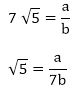We know that √5 is an irrational number
Here we see that √5 is a rational number which is a contradiction.
(ii) Let us assume that 6+√2 is rational. Then, there exist positive co primes a and b such that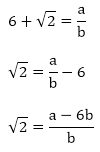Here we see that √2 is a rational number which is a contradiction as we know that √2 is an irrational number
Hence 6 + √2 is an irrational number
(iii) Let us assume that 3 - √5 is rational. Then, there exist positive co primes a and b such that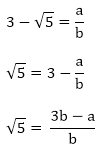Here we see that √5 is a rational number which is a contradiction as we know that √5 is an irrational number
Hence 3 - √5 is an irrational number.

Question: 2
Prove that the following numbers are irrationals.
(i) 2√7
(ii) 3/(2√5)
(iii) 4 + √2
(iv)  5√2
Solution:
(i) Let us assume that 2√7 is rational. Then, there exist positive co primes a and b such that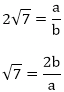√7 is rational number which is a contradiction
Hence 2√7 is an irrational number

(ii) Let us assume that 3/(2√5) is rational. Then, there exist positive co primes a and b such that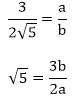√5 is rational number which is a contradiction
Hence 3/(2√5) is irrational.

(iii) Let us assume that 3/(2√5) is rational. Then, there exist positive co primes a and b such that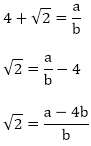√2 is rational number which is a contradiction
Hence 4+ √2 is irrational.

(iv) Let us assume that 5√2 is rational. Then, there exist positive co primes a and b such that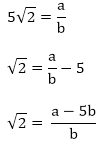√2 is rational number which is a contradiction
Hence 5√2 is irrational

Question: 3
Show that 2 - √3 is an irrational number.
Solution:
Let us assume that 2 - √3 is rational. Then, there exist positive co primes a and b such that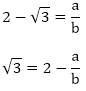Here we see that √3 is a rational number which is a contradiction
Hence 2- √3 is irrational

Question: 4
Show that 3 + √2 is an irrational number.
Solution:
Let us assume that 3 + √2 is rational. Then, there exist positive co primes a and b such that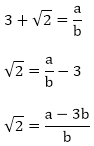Here we see that √2 is a irrational number which is a contradiction
Hence 3 + √2 is irrational

Question: 5
Prove that 4 - 5√2 is an irrational number.
Solution:
Let us assume that 4 - 5√2 is rational. Then, there exist positive co primes a and b such that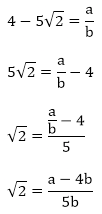This contradicts the fact that √2 is an irrational number
Hence 4 – 5√2 is irrational

Question: 6
Show that 5 - 2√3 is an irrational number.
Solution:
Let us assume that 5 -2√3 is rational. Then, there exist positive co primes a and b such that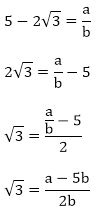This contradicts the fact that √3 is an irrational number
Hence 5 – 2√3 is irrational

Question: 7
Prove that 2√3 - 1 is an irrational number.
Solution:
Let us assume that 2√3 – 1 is rational. Then, there exist positive co primes a and b such that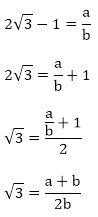This contradicts the fact that √3 is an irrational number
Hence 5 - 2√3 is irrational

Question: 8
Prove that 2 - 3√5 is an irrational number.
Solution:
Let us assume that 2 - 3√5 is rational. Then, there exist positive co primes a and b such that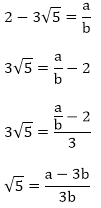This contradicts the fact that √5 is an irrational number
Hence 2 - 3√5 is irrational

Question: 9
Prove that √5 + √3 is irrational.
Solution:
Let us assume that √5 + √3 is rational. Then, there exist positive co primes a and b such that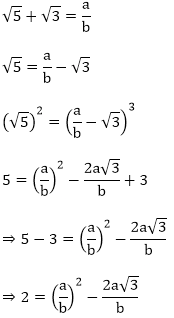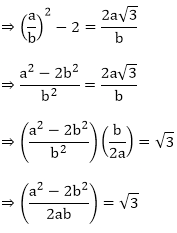Here we see that √3 is a rational number which is a contradiction as we know that √3 is an irrational number
Hence √5 + √3 is an irrational number

Question: 10
Prove that √3 + √4 is irrational.
Solution:
Let us assume that √3 + √4 is rational. Then, there exist positive co primes a and b such that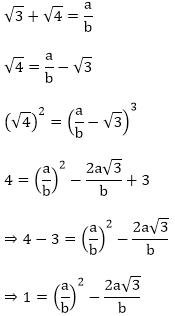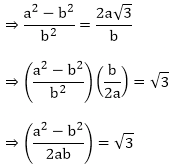Here we see that √3 is a rational number which is a contradiction as we know that √3 is an irrational number
Hence √3 + √4   is an irrational number

Question: 11
Prove that for any prime positive integer p, √p is an irrational number.
Solution:
Let us assume that √p is rational. Then, there exist positive co primes a and b such that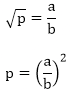⇒ pb2 = a2
⇒ p|a2 ⇒ p|a2
⇒ p|a ⇒ p|a
⇒ a = pc for some positive integer c ⇒ a = pc for some positive integer c

b2p = a2
⇒ b2p = p2c2 ( ∵ a = pc)
⇒ p|b2 (since p|c2p) ⇒ p|b2(since p|c2p)
⇒ p|b ⇒ p|a and p|b
This contradicts the fact that a and b are co primes
Hence √p is irrational

Question: 12
If p, q are prime positive integers, prove that √p + √q is an irrational number.
Solution:
Let us assume that √p + √q is rational. Then, there exist positive co primes a and b such that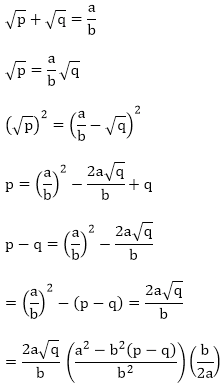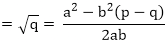Here we see that √q is a rational number which is a contradiction as we know that √q is an irrational number
Hence √p + √q  is an irrational number

Exercise - 1.6

Question: 1
Without actually performing the long division, state whether the following rational numbers will have a terminating decimal expansion or a non-terminating repeating decimal expansion.
(i)  23/8
(ii) 125/441
(iii) 35/50
(iv) 77/210
(v)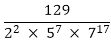Solution:
(i) The given number is 23/8
Here, 8 = 23 and 2 is not a factor of 23.
So, the given number is in its simplest form.
Now, 8 = 23 is of the form 2m × 5n, where m = 3 and n = 0.
So, the given number has a terminating decimal expansion.
(ii) The given number is 125/441
Here, 441 = 32 × 72 and none of 3 and 7 is a factor of 125.
So, the given number is in its simplest form.
Now, 441 = 32 × 72 is not of the form 2m × 5n
So, the given number has a non-terminating repeating decimal expansion.
(iii) The given number is 35/50 and HCF (35, 50) = 5.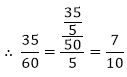Here, 7/10 is in its simplest form.
Now, 10 = 2 × 5 is of the form 2m × 5n, where in = 1 and n = 1.
So, the given number has a terminating decimal expansion.
(iv) The given number is 77/210 and HCF (77, 210) = 7.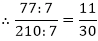Here, 11/30 is in its simplest form. 30
Now, 30 = 2 × 3 × 5 is not of the form 2m × 5n.
So, the given number has a non-terminating repeating decimal expansion.
(v) The given number is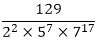Clearly, none of 2, 5 and 7 is a factor of 129.
So, the given number is in its simplest form.

Question: 2
Write down the decimal expansions of the following rational numbers by writing their denominators in the form of 2m × 5n, where m, and n, are the non- negative integers.
(i)  ⅜
(ii)  13/125
(iii)  7/80
(iv) 14588/625
(v)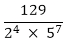Solution.
(i) The given number is ⅜
Clearly, 8 = 23 is of the form 2m × 5n, where m = 3 and n = 0.
So, the given number has terminating decimal expansion.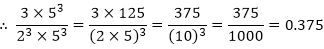(ii) The given number is 13/125
Clearly, 125 = 53 is of the form 2m × 5n, where m = 0 and n = 3.
So, the given number has terminating decimal expansion.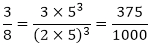(iii) The given number is 7/80.
Clearly, 80 = 24 × 5 is of the form 2m × 5n, where m = 4 and n = 1.
So, the given number has terminating decimal expansion.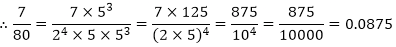(iv) The given number is 14588/625
Clearly, 625 = 54 is of the form 2m × 5n, where m = 0 and n = 4.
So, the given number has terminating decimal expansion?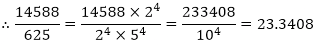(v) The given number is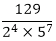Clearly, 22 × 57 is of the form 2m × 5n, where in = 2 and n = 7.
So, the given number has terminating decimal expansion?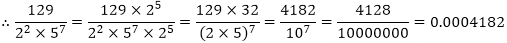Question: 3
What can you say about the prime factorization of the denominators of the following rational?
(i) 43.123456789
(ii)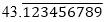(iii)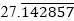(iv) 0.120120012000120000
Solution.
(i) Since 43.123456789 has terminating decimal expansion. So, its denominator is of the form 2m × 5n, where m, n are non-negative integers.
(ii) Since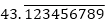has non-terminating decimal expansion. So, its denominator has factors other than 2 or 5.
(iii) Since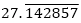has non-terminating decimal expansion. So, its denominator has factors other than 2 or 5.
(iv) Since 0.120120012000120000 … has non-terminating decimal expansion. So, its denominator has factors other than 2 or 5.

Offer running on EduRev: Apply code STAYHOME200 to get INR 200 off on our premium plan EduRev Infinity!

## Mathematics (Maths) Class 10

59 videos|362 docs|103 tests

,

,

,

,

,

,

,

,

,

,

,

,

,

,

,

,

,

,

,

,

,

;